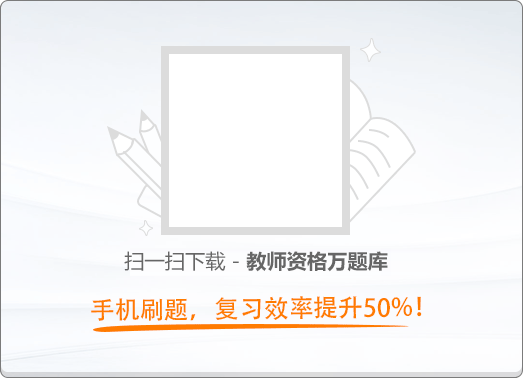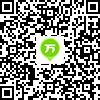120:002018年3月《幼儿保教知识与能力》真题

1
(单项选择题)

• A.

促进幼儿智力发展

• B.

促进幼儿身心的快速发展

• C.

促进幼儿社会性发展

• D.

促进幼儿身心全面和谐发展

• A
• B
• C
• D

2
(单项选择题)

• A.

要求幼儿用胸腔式联合呼吸法唱歌

• B.

鼓励幼儿用最响亮的声音唱歌

• C.

鼓励幼儿唱八度以上音域的歌唱

• D.

要求幼儿用自然声音唱歌

• A
• B
• C
• D

3
(单项选择题)

• A.

羞愧

• B.

伤心

• C.

害怕

• D.

生气

• A
• B
• C
• D

4
(单项选择题)

• A.

幼儿的情绪表达能力

• B.

幼儿的社会交往能力

• C.

幼儿的规则意识

• D.

幼儿的思维发展水平

• A
• B
• C
• D

5
(单项选择题)

• A.

信任感

• B.

主动性

• C.

自主性

• D.

自我同一性

• A
• B
• C
• D

6
(单项选择题)

• A.

能概括不同声音产生的条件

• B.

对声音产生兴趣，感受不同的声音

• C.

能描述出玩具是怎么发声的

• D.

能描述不同玩具发声特点

• A
• B
• C
• D

7
(单项选择题)

• A.

引导幼儿感知常见事物的大小、高矮、粗细等

• B.

引导幼儿识别常见食物的形状

• C.

和幼儿一起手口一致点数物体，说出总数

• D.

为幼儿提供按数取物的机会

• A
• B
• C
• D

8
(单项选择题)

• A.

发展幼儿的艺术技能

• B.

培养幼儿的艺术感受和表达能力

• C.

丰富幼儿的艺术知识

• D.

拓展幼儿的逻辑思维能力

• A
• B
• C
• D

9
(单项选择题)

• A.

讲授制

• B.

五指活动

• C.

感官教育

• D.

艺友制

• A
• B
• C
• D

10
(单项选择题)

• A.

儿童的深度知觉

• B.

儿童的计数能力

• C.

儿童的自我中心性

• D.

儿童的守恒能力

• A
• B
• C
• D